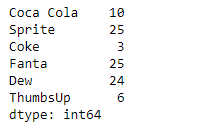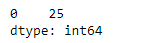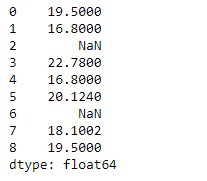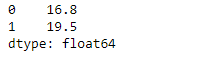# Python | Pandas Series.mode()

• Last Updated : 11 Feb, 2019

Pandas series is a One-dimensional ndarray with axis labels. The labels need not be unique but must be a hashable type. The object supports both integer- and label-based indexing and provides a host of methods for performing operations involving the index.

Pandas` Series.mode()` function return the mode of the underlying data in the given Series object. This function always returns Series even if only one value is returned.

Attention geek! Strengthen your foundations with the Python Programming Foundation Course and learn the basics.

To begin with, your interview preparations Enhance your Data Structures concepts with the Python DS Course. And to begin with your Machine Learning Journey, join the Machine Learning - Basic Level Course

Syntax: Series.mode(dropna=True)

Parameter :
dropna : Don’t consider counts of NaN/NaT

Returns : modes : Series

Example #1: Use `Series.mode()` function to find the mode of the given series object.

 `# importing pandas as pd``import` `pandas as pd`` ` `# Creating the Series``sr ``=` `pd.Series([``10``, ``25``, ``3``, ``25``, ``24``, ``6``])`` ` `# Create the Index``index_ ``=` `[``'Coca Cola'``, ``'Sprite'``, ``'Coke'``, ``'Fanta'``, ``'Dew'``, ``'ThumbsUp'``]`` ` `# set the index``sr.index ``=` `index_`` ` `# Print the series``print``(sr)`

Output :Now we will use `Series.mode()` function to find the mode of the given series object.

 `# return the mode``result ``=` `sr.mode()`` ` `# Print the result``print``(result)`

Output :As we can see in the output, the `Series.mode()` function has successfully returned the mode of the given series object.

Example #2: Use `Series.mode()` function to find the mode of the given series object. The given series object contains some missing values.

 `# importing pandas as pd``import` `pandas as pd`` ` `# Creating the Series``sr ``=` `pd.Series([``19.5``, ``16.8``, ``None``, ``22.78``, ``16.8``, ``20.124``, ``None``, ``18.1002``, ``19.5``])`` ` `# Print the series``print``(sr)`

Output :Now we will use `Series.mode()` function to find the mode of the given series object.

 `# return the mode``result ``=` `sr.mode()`` ` `# Print the result``print``(result)`

Output :As we can see in the output, the `Series.mode()` function has successfully returned the mode of the given series object.

My Personal Notes arrow_drop_up